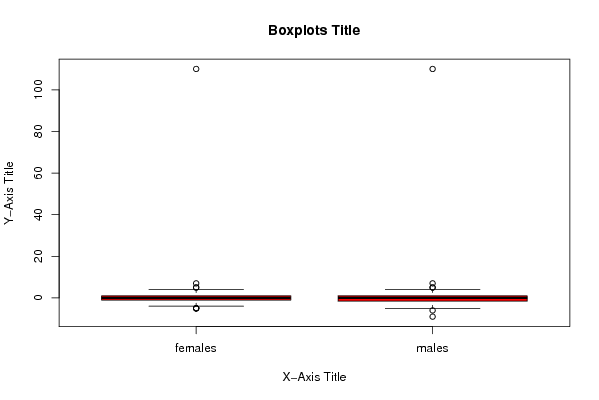## Free Statistics

of Irreproducible Research!

Author's title
Author*The author of this computation has been verified*
R Software Modulerwasp_Tests to Compare Two Means.wasp
Title produced by softwareT-Tests
Date of computationWed, 01 Sep 2010 14:22:17 +0000
Cite this page as followsStatistical Computations at FreeStatistics.org, Office for Research Development and Education, URL https://freestatistics.org/blog/index.php?v=date/2010/Sep/01/t1283350926nsans7700v1wvgi.htm/, Retrieved Tue, 21 Mar 2023 08:03:58 +0000
Statistical Computations at FreeStatistics.org, Office for Research Development and Education, URL https://freestatistics.org/blog/index.php?pk=79506, Retrieved Tue, 21 Mar 2023 08:03:58 +0000
QR Codes:Original text written by user:
IsPrivate?No (this computation is public)
User-defined keywords
Estimated Impact186
Family? (F = Feedback message, R = changed R code, M = changed R Module, P = changed Parameters, D = changed Data)
-     [Two-Way ANOVA] [two-way anova wit...] [2010-05-26 17:02:24] [98fd0e87c3eb04e0cc2efde01dbafab6]
- R PD  [Two-Way ANOVA] [ANOVA with good l...] [2010-05-28 23:09:47] [98fd0e87c3eb04e0cc2efde01dbafab6]
- R       [Variability] [ANOVA with better...] [2010-05-29 09:47:12] [98fd0e87c3eb04e0cc2efde01dbafab6]
- R         [Variability] [ANOVA with better...] [2010-05-29 09:54:40] [98fd0e87c3eb04e0cc2efde01dbafab6]
- RMPD        [T-Tests] [] [2010-09-01 14:01:06] [d67ed87d94f8374cf5eb71740c29a070]
-   PD            [T-Tests] [] [2010-09-01 14:22:17] [14a8bfb44cf78e94acc31dd768e0bbfd] [Current]
Feedback Forum

Post a new message
Dataseries X:
7	0
-1	-2
0	0
110	-1
-1	-4
0	0
-5	1
-1	-5
0	-9
0	1
0	-5
-5	-1
0	-4
2	1
-2	2
-2	3
0	-1
0	0
5	2
2	-2
1	0
4	-2
-1	3
-2	-3
0	1
-1	-5
3	1
1	-1
1	5
2	-1
1	-1
1	0
1	-5
-1	-1
0	4
-5	0
-1	-1
1	-1
1	-1
5	-2
0	-4
0	2
-2	-2
1	-2
0	1
-1	2
0	0
0	-6
0	0
0	0
0	0
-1	2
2	-1
1	-1
2	1
0	-4
4	-2
-2	-1
-1	-1
0	2
0	-2
0	2
0	-4
0	1
0	-2
1	1
3	1
3	0
0	-2
2	3
-1	-1
-2	-1
-1	0
-2	2
0	0
0	1
4	-5
3	3
-1	3
0	3
2	-1
4	-2
1
1
0
1
1
0
1
0
3
4
0
5
4
1
0
0
1


 Summary of computational transaction Raw Input view raw input (R code) Raw Output view raw output of R engine Computing time 1 seconds R Server 'Gwilym Jenkins' @ 72.249.127.135

\begin{tabular}{lllllllll}
\hline
Summary of computational transaction \tabularnewline
Raw Input & view raw input (R code)  \tabularnewline
Raw Output & view raw output of R engine  \tabularnewline
Computing time & 1 seconds \tabularnewline
R Server & 'Gwilym Jenkins' @ 72.249.127.135 \tabularnewline
\hline
\end{tabular}
%Source: https://freestatistics.org/blog/index.php?pk=79506&T=0

[TABLE]
[ROW][C]Summary of computational transaction[/C][/ROW]
[ROW][C]Raw Input[/C][C]view raw input (R code) [/C][/ROW]
[ROW][C]Raw Output[/C][C]view raw output of R engine [/C][/ROW]
[ROW][C]Computing time[/C][C]1 seconds[/C][/ROW]
[ROW][C]R Server[/C][C]'Gwilym Jenkins' @ 72.249.127.135[/C][/ROW]
[/TABLE]
Source: https://freestatistics.org/blog/index.php?pk=79506&T=0

Globally Unique Identifier (entire table): ba.freestatistics.org/blog/index.php?pk=79506&T=0

As an alternative you can also use a QR Code:

The GUIDs for individual cells are displayed in the table below:

 Summary of computational transaction Raw Input view raw input (R code) Raw Output view raw output of R engine Computing time 1 seconds R Server 'Gwilym Jenkins' @ 72.249.127.135

 T-Test Mean1 1.39394 Mean2 0.73737 T Statistic 0.40873 P-value 0.34159 Lower Confidence Limit -1.9982 Upper Confidence Limit Inf

\begin{tabular}{lllllllll}
\hline
T-Test \tabularnewline
Mean1 & 1.39394 \tabularnewline
Mean2 & 0.73737 \tabularnewline
T Statistic & 0.40873 \tabularnewline
P-value & 0.34159 \tabularnewline
Lower Confidence Limit & -1.9982 \tabularnewline
Upper Confidence Limit & Inf \tabularnewline
\hline
\end{tabular}
%Source: https://freestatistics.org/blog/index.php?pk=79506&T=1

[TABLE]
[ROW][C]T-Test[/C][/ROW]
[ROW][C]Mean1[/C][C]1.39394[/C][/ROW]
[ROW][C]Mean2[/C][C]0.73737[/C][/ROW]
[ROW][C]T Statistic[/C][C]0.40873[/C][/ROW]
[ROW][C]P-value[/C][C]0.34159[/C][/ROW]
[ROW][C]Lower Confidence Limit[/C][C]-1.9982[/C][/ROW]
[ROW][C]Upper Confidence Limit[/C][C]Inf[/C][/ROW]
[/TABLE]
Source: https://freestatistics.org/blog/index.php?pk=79506&T=1

Globally Unique Identifier (entire table): ba.freestatistics.org/blog/index.php?pk=79506&T=1

As an alternative you can also use a QR Code:

The GUIDs for individual cells are displayed in the table below:

 T-Test Mean1 1.39394 Mean2 0.73737 T Statistic 0.40873 P-value 0.34159 Lower Confidence Limit -1.9982 Upper Confidence Limit Inf

 Standard Deviations Variable 1 11.22026 Variable 2 11.38257

\begin{tabular}{lllllllll}
\hline
Standard Deviations \tabularnewline
Variable 1 & 11.22026 \tabularnewline
Variable 2 & 11.38257 \tabularnewline
\hline
\end{tabular}
%Source: https://freestatistics.org/blog/index.php?pk=79506&T=2

[TABLE]
[ROW][C]Standard Deviations[/C][/ROW]
[ROW][C]Variable 1[/C][C]11.22026[/C][/ROW]
[ROW][C]Variable 2[/C][C]11.38257[/C][/ROW]
[/TABLE]
Source: https://freestatistics.org/blog/index.php?pk=79506&T=2

Globally Unique Identifier (entire table): ba.freestatistics.org/blog/index.php?pk=79506&T=2

As an alternative you can also use a QR Code:

The GUIDs for individual cells are displayed in the table below:

 Standard Deviations Variable 1 11.22026 Variable 2 11.38257PNG link Postscript link PDF link

Parameters (Session):
par1 = greater ; par2 = 1 ; par3 = 2 ; par4 = T-Test ; par5 = unpaired ; par6 = 0.0 ; par7 = 0.95 ; par8 = TRUE ;
Parameters (R input):
par1 = greater ; par2 = 1 ; par3 = 2 ; par4 = T-Test ; par5 = unpaired ; par6 = 0.0 ; par7 = 0.95 ; par8 = TRUE ;
R code (references can be found in the software module):
par2 <- as.numeric(par2)par3 <- as.numeric(par3)par4 <- as.character(par4)par5 <- as.character(par5)par6 <- as.numeric(par6)par7 <- as.numeric(par7)par8 <- as.logical(par8)if ( par5 == 'unpaired') paired <- FALSE else paired <- TRUEx <- t(y)if(par8){bitmap(file='test1.png')(r<-boxplot(x ,xlab=xlab,ylab=ylab,main=main,notch=FALSE,col=2))dev.off()}load(file='createtable')if( par4 == 'Wilcoxon-Mann_Whitney'){a<-table.start()a <- table.row.start(a)a <- table.element(a,'Wilcoxon Test',3,TRUE)a <- table.row.end(a)a <- table.row.start(a)a <- table.element(a,'',1,TRUE)a <- table.element(a,'Statistic',1,TRUE)a <- table.element(a,'P-value',1,TRUE)a <- table.row.end(a)W <- wilcox.test(x[,par2],x[,par3],alternative=par1, paired = paired)a<-table.row.start(a)a<-table.element(a,'Wilcoxon Test',1,TRUE)a<-table.element(a,W$statistic[])a<-table.element(a,round(W$p.value, digits=5) )a<-table.row.end(a)a<-table.end(a)table.save(a,file='mytable.tab')}if( par4 == 'T-Test'){T <- t.test(x[,par2],x[,par3],alternative=par1, paired=paired, mu=par6, conf.level=par7)a<-table.start()a <- table.row.start(a)a <- table.element(a,'T-Test',3,TRUE)a <- table.row.end(a)if(paired){a <- table.row.start(a)a <- table.element(a,'Difference: Mean1 - Mean2',1,TRUE)a<-table.element(a,round(T$estimate, digits=5) )a <- table.row.end(a)}if(!paired){a <- table.row.start(a)a <- table.element(a,'Mean1',1,TRUE)a<-table.element(a,round(T$estimate, digits=5) )a <- table.row.end(a)a <- table.row.start(a)a <- table.element(a,'Mean2',1,TRUE)a<-table.element(a,round(T$estimate, digits=5) )a <- table.row.end(a)}a <- table.row.start(a)a <- table.element(a,'T Statistic',1,TRUE)a<-table.element(a,round(T$statistic, digits=5) )a <- table.row.end(a)a <- table.row.start(a)a <- table.element(a,'P-value',1,TRUE)a<-table.element(a,round(T$p.value, digits=5) )a <- table.row.end(a)a <- table.row.start(a)a <- table.element(a,'Lower Confidence Limit',1,TRUE)a<-table.element(a,round(T$conf.int, digits=5) )a <- table.row.end(a)a<-table.row.start(a)a <- table.element(a,'Upper Confidence Limit',1,TRUE)a<-table.element(a,round(T\$conf.int, digits=5) )a <- table.row.end(a)a<-table.end(a)table.save(a,file='mytable.tab')}a<-table.start()a <- table.row.start(a)a <- table.element(a,'Standard Deviations',3,TRUE)a <- table.row.end(a)a <- table.row.start(a)a <- table.element(a,'Variable 1',1,TRUE)a<-table.element(a,round(sd(x[,par2]), digits=5) )a <- table.row.end(a)a <- table.row.start(a)a <- table.element(a,'Variable 2',1,TRUE)a<-table.element(a,round(sd(x[,par3]), digits=5) )a <- table.row.end(a)a<-table.end(a)table.save(a,file='mytable1.tab')